# Optical Signal-To-Noise Ratio (OSNR)

The ASE noise added by each amplifier to the signal reduces the SNR of the amplified signal. The performance of a lightwave system is dictated by the electrical SNR obtained after the signal has been converted into an electric form using a photodetector. However, the concept of optical SNR, defined as the ratio of optical power to the ASE power (within a certain bandwidth), is also useful as it can provide design guidelines. In this section we focus on the optical SNR and consider electrical SNR in the next section.

#### 1. Lumped Amplification

In a chain of cascaded lumped amplifiers, ASE accumulates from amplifier to amplifier and can build up to high levels. The ASE power added by any amplifier is attenuated in succeeding fiber sections, but it is also amplified by all following amplifiers. The net effect is that one can simply add the ASE powers of all amplifiers at the end of a fiber link. Assuming that all amplifiers are spaced apart by the same distance LA and are operated with the same gain G = exp(αLA), the total ASE power for a chain of NA amplifiers is given bywhere the factor of 2 takes into account the unpolarized nature of ASE and Δν0 is the bandwidth of the optical filter.

Clearly, ASE power can become quite large for large values of G and NA. A side effect of high ASE levels is that, at some point, ASE begins to saturate amplifiers. Then, signal power is reduced while, at the same time, noise power keeps on increasing, resulting in severe degradation of the SNR. The ASE power can be controlled, and SNR can be improved, by reducing the amplifier spacing LA. At first sight, this approach appears counterintuitive since it increases the number NA of amplifiers. However, noting that NA = LT/LA = αLT/lnG for a link of total length LT, we find thatscales with G as (G-1)/lnG, and can be lowered by simply reducing the gain G of each amplifier. Thus, increasing the number amplifiers while reducing their separation is an effective technique for controlling ASE noise. This feature explains why distributed amplification helps to improve the performance of a lightwave system.

In practice, one may want to minimize the number of lumped amplifiers for economical reasons. In this situation, the system should be designed with an amplifier spacing that is relatively large but still small enough to operate the system reliably and to realize a target value of optical SNR. From the equation above, the optical SNR can be written as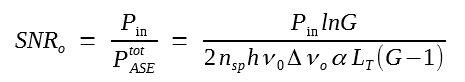where Pin is the input average power. The following figure shows the total system length LT as a function of LA for several values of input powers using α = 0.2 dB/km, nsp = 1.6, Δνo = 100 GHz, and assuming that an optical SNR of 20 is required for the system to function properly.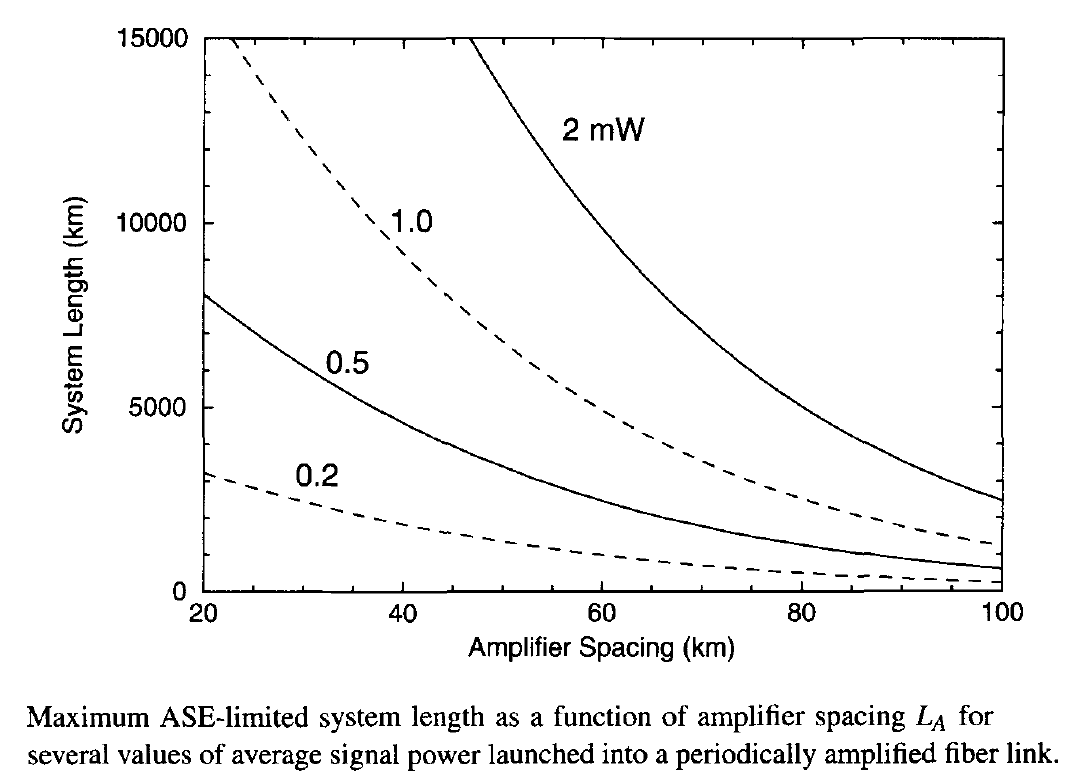For a given input power, amplifier spacing LA becomes smaller as the system length increases. Although amplifier spacing can be improved by increasing the input power Pin, in practice, the maximum power that can be launched is limited by the onset of various nonlinear effects discussed before. Typically, Pin is limited to close to 1 mW. At such power levels, LA should be in the range of 40 to 50 km for submarine lightwave systems with lengths of 6,000 km or more, but it can be increased to 80 km or so for terrestrial systems with link lengths under 3,000 km.

#### 2. Distributed Amplification

In the case of distributed amplification, pump power is injected periodically at pump stations spaced apart by LA and is chosen such that the signal power recovers at each pump station to its input value, that is, Ps(nLA) = Pin. One can use an approach similar to that employed for lumped amplifiers for calculating the optical SNR and finding the optimum pump-station spacing. The optical SNR of the amplified signal in this case takes the form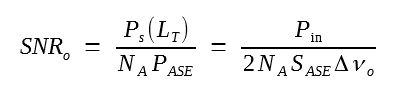where LT = NALA is the link length, NA is the number of pump stations, and SASE is given below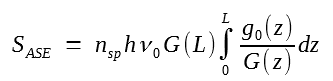A new feature of distributed amplification is that the pump power can be injected in the forward, backward, or both directions. Since the functional form of gain g(z) depends on the pumping scheme, and SASE depends on g(z), we can control the optical SNR to some extent by adopting a suitable pumping scheme. The figure below shows how (a) ASE spectral density and (b) optical SNR vary with the net gain G(L) for several different pumping schemes, assuming that a 1-mW input signal is transmitted through a 100-km-long, bidirectionally pumped, distributed Raman amplifier.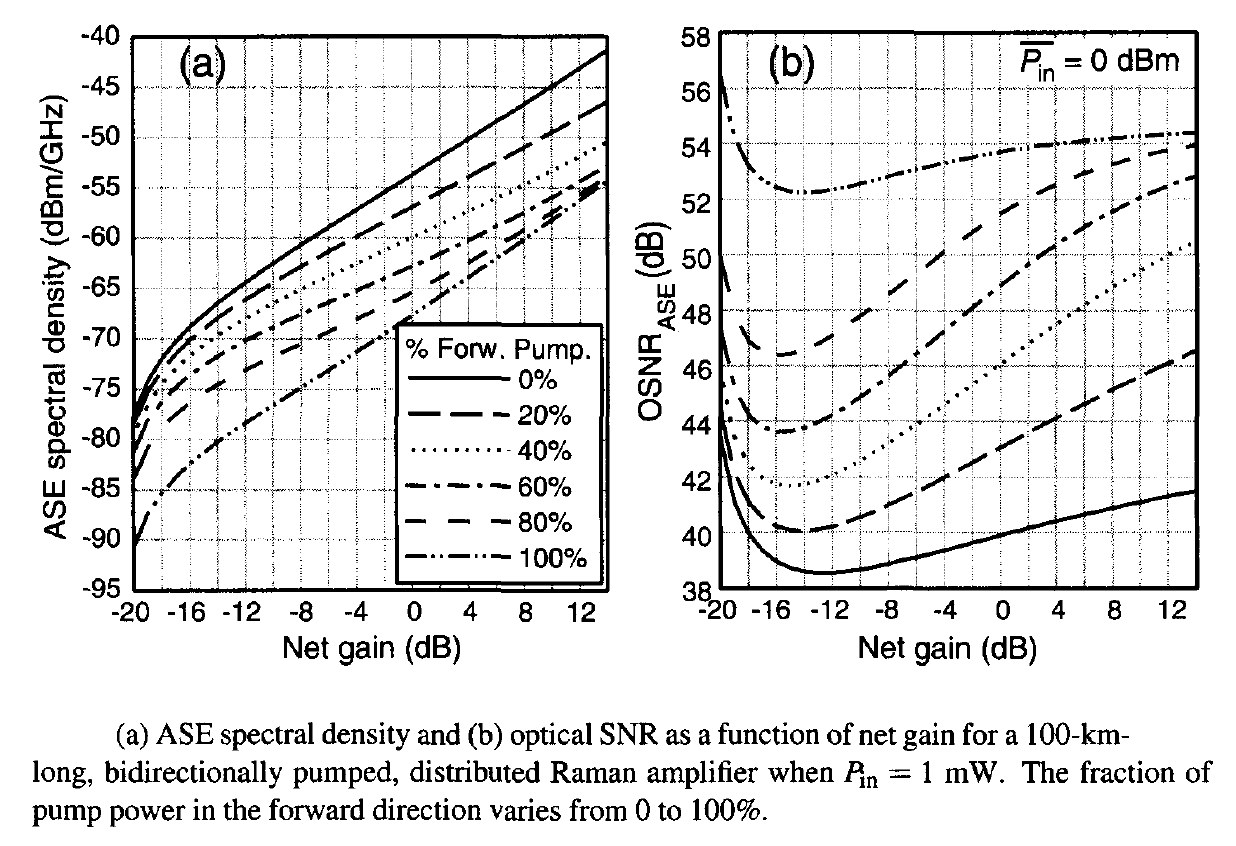The fraction of forward pumping varies from 0 to 100%. Fiber losses are 0.26 and 0.21 dB/km at the pump and signal wavelengths, respectively. Other parameters used were nsp = 1.13, hν0 = 0.8 eV, and gR = 0.68 W-1/km. The optical SNR is highest in the case of purely forward pumping (about 54 dB or so), but it degrades by as much as 15 dB as the fraction of backward pumping is increased from 0 to 100%. This can be understood by noting that the ASE generated near the input end experiences accumulated losses over the full length of the fiber in the case of forward pumping, whereas it experiences only a fraction of such losses in the case of backward pumping.

Figure (b) above shows the optical SNR for a single 100-km-long section but it illustrates clearly the benefits of distributed amplification. If NA such sections are employed to form a long-haul fiber link, SNR is reduced by a factor of NA as seen from the SNRo equation above. Even when NA = 100 and the total link length LT = 10,000 km, SNRo remains larger than 20 dB. Such high values of optical SNR are difficult to maintain for long fiber links when EDFAs are used.